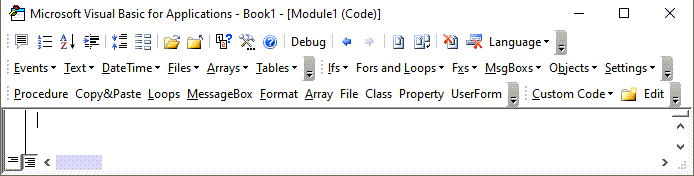# VBA CDbl Function – Convert to Double

This tutorial will demonstrate how to use the CDbl VBA function to convert expressions to double data type.

## CDbl Function

### VBA CDbl Convert Expression to Integer

The VBA CDbl function can be used to convert expressions to double data type inside VBA code.

``````Sub CDblExample_1()
MsgBox CDbl(12.345)     'Result is: 12.345
MsgBox CDbl(-124)       'Result is: -124
MsgBox CDbl(0.000034)   'Result is: 0.000034
MsgBox CDbl(-12.000034) 'Result is: -12.000034
End Sub
``````

### VBA CDbl Converting String to Double

The VBA CDbl function can be used to convert strings to doubles if the characters in the string have a meaning as numbers.

``````Sub CDblExample_2()
Dim StrEx As String
StrEx = "112"
MsgBox CDbl(StrEx)
'Result is: 112

StrEx = "0.0003"
MsgBox CDbl(StrEx)
'Result is: 0.0003

StrEx = "11,00002"
MsgBox CDbl(StrEx)
'Result is: 1100002   , is ignored

StrEx = "\$112"
MsgBox CDbl(StrEx)
'Result is: 112        \$ is ignored
End Sub
``````

### VBA CDbl Run-Time Error 13 Type Mismatch

Using VBA CDbl function with strings that contain non-numerical characters or characters that don’t have meaning in numerical context will result in a Run-Time error ’13’: Type mismatch.

``````Sub CDblExample_3()
'The code below will result in an ERROR message
'CDbl cant handle non numerical characters
Dim StrEx As String
StrEx = "Ab13"
MsgBox CDbl(StrEx)
End Sub
``````

### VBA CDbl Regional Settings

VBA CDbl function has different behavior converting strings with comma or dot.  It uses the Regional Settings of the operating system for decimal separator and digit separator.

``````Sub CDblExample_4()
Dim StrEx As String
StrEx = "1,9"
MsgBox CDbl(StrEx)
'If Regional settings have , as a grouping separator then
'Result is: 19
'If Regional settings have , as a decimal separator then
'Result is: 1,9

StrEx = "1.9"
MsgBox CDbl(StrEx)
'If Regional settings have . as a grouping separator then
'Result is: 19
'If Regional settings have . as a decimal separator then
'Result is: 1.9
End Sub
``````

### VBA CDbl Converting Booleans to Doubles

VBA CDbl function can convert boolean variables to doubles. If the evaluated expression is true the resulting double is -1 and if the evaluated expression is false the resulting double is 0.

``````Sub CDblExample_5()
Dim BoolEx As Boolean
BoolEx = True
MsgBox CDbl(BoolEx)  'Result is: -1
MsgBox CDbl(2 = 2)   'Result is: -1

BoolEx = False
MsgBox CDbl(BoolEx)  'Result is: 0
MsgBox CDbl(1 = 2)   'Result is: 0
End Sub
``````

Stop searching for VBA code online. Learn more about AutoMacro - A VBA Code Builder that allows beginners to code procedures from scratch with minimal coding knowledge and with many time-saving features for all users!### VBA CDbl Converting Dates to Doubles

VBA CDbl function can convert a date variable to an integer. The returned value is the internal number used by excel for date storage rounded.

``````Sub CDblExample_6()
Dim DateEx As Date
DateEx = #2/3/1940#
MsgBox CDbl(DateEx)
'Result is: 14644
DateEx = #8/7/1964 10:41:00 PM#
MsgBox CDbl(DateEx)
'Result is: 23596.9451388889
DateEx = #3/7/1934 11:32:04 AM#
MsgBox CDbl(DateEx)
'Result is: 12485.4806018519
End Sub
``````